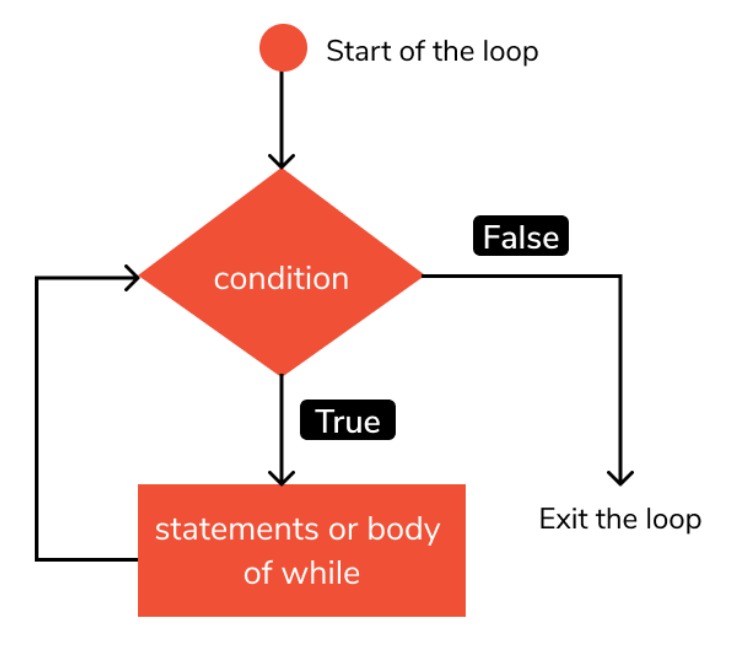# Python While And While-else Loop

Python is a high-level, interpreted programming language, designed and developed by Guido van Rossum, and is one of the most popular programming languages present in the software industry.

One of the most important but often overlooked concepts in python is that of while and while-else loops. Let us have a look at them in detail.

## What are while loops in Python?

In python, while loops are used for the purpose of repeated execution of a given statement in a conditional manner, that is, as long as a particular condition is satisfied.

The statements are stopped being executed as soon as the specified condition becomes violated, that is, returns False.

If the condition returns False before the while loop is executed even once, the body of the while loop is not executed and is skipped.

The following flowchart will help you to understand the concept of while loops in a better manner.### Python While Loop Syntax

The syntax of while loops are as follows. The <expression> refers to the boolean condition that decides if the statements in the body of the while loop will be executed for that iteration or not.

Note that the statements to be executed per iteration are added from the next line onwards with an indentation.

The keyword used here is while. A colon is also present at the end of the first line.

``````while <expression>:
<statements to be executed per iteration>``````

## Python While loop Example

In this example, we shall use a variable that contains data of integer type. We name this variable as i.

The condition that decides whether the body of the while loop is i<3 which means that as long as the value of i is less than 3, the body of the while loop is executed.

Inside the body of the while loop, we first print the value of i and then in the next line increment it using i += 1. This means the body of the while loop will be executed for a total of 3 times when i is 0,1 and 2 respectively.

As soon as the value of i becomes 3 after incrementing, the expression i<3 returns False when evaluated and the while loop stops executing.

Let us look at the python code and corresponding output for this example -

``````# While  loop example in python
i=0
while i<3:
Â Â print(i)
Â Â i += 1``````

Output

0

1

2

## Nested while loop in Python

While loop declared inside another while loop forms a nested loop.  A nested loop is nothing more than that a loop contains another loop in its body.

In this method, we shall use variables that contain data of integer type. We name these variables as i and j.

Here, we have two while loops, the outer loop, and the inner loop. The condition that decides whether the body of the outer while loop is executed is i<2 which means that as long as the value of i is less than 2, the body of the outer while loop is executed.

Inside the body of the outer while loop, we have a variable j initialized to 0 and another loop, the inner while loop with the condition j<2.

In this, we first print the value of j and then in the next line increment it using j += 1.

So for each execution of the outer loop, the inner loop is executed twice and the outer loop itself is executed twice which totals to 4 lines being printed.

Let us look at the python code and corresponding output for this example.

``````# Nested while loop in python
i=0
while i<2:
Â Â j=0
Â Â while j<2:
Â Â Â Â print(j)
Â Â Â Â j += 1``````

Output

0

1

0

1

## Infinite while loop in Python

Let us say that for some reason, we did not add the line i += 1 that is used to increment the value in the variable i after each iteration.

This case is almost similar to a normal while loop but one key difference is that the condition of the while loop never becomes False.

This means that the while loop will continuously keep on running until some external interrupt stops the execution of the program.

This is a very dangerous situation that is often overlooked but often causes problems during debugging.

Let us look at the python code and corresponding output for this example:

``````# Infinite while loop in python
i=0
while i<3:
Â Â print(i)``````

Output

0

0

0….infinitely

## While-else loops in Python

Another statement we can use along with while loops is the else statement and in such cases, it is known as the while-else loop.

The statements specified by the else statement are executed once the while loop finishes execution.

For this, we use the else keyword. Let us look at the python code and corresponding output for this example.

``````# While-else loop in python
i = 0
while i<3:
Â Â print(i)
Â Â i+=1
else:
Â Â print("While loop finished execution")``````

Output

0

1

2

While loop finished execution

## Single statement while loops in Python

A special case of while loops are when the body of the while loop contains only one statement. Here that statement is print(i).

This case is the same as the case of infinite while loop where the condition of the while loop never becomes False. This means that the while loop will continuously keep on running until some external interrupt stops the execution of the program.

Let us look at the python code and corresponding output for this example.

``````# Single statement while loop in python
i = 0
while i<3: print(i)``````

Output

0

0

0….infinitely

## Conclusion

In this topic, we have learned the use and advantages of while and while-else loops in a Python program, following a running example of a simple iterative printing program, thus giving us an intuition of how this concept could be applied in real-world situations. Feel free to reach out to info.javaexercise@gmail.com in case of any suggestions

Trending